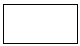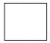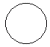Name: ___________________Date:___________________

 Email us to get an instant 20% discount on highly effective K-12 Math & English kwizNET Programs!

### Grade 1 - Mathematics5.1 Name the Shapes

 There are many shapes that we see around us in our daily lives Two dimensional:Circle : A shape which is exactly round. Triangle: A shape that has 3 sides. Square : A shape that has 4 sides. Rectangle: A shape that has 4 sides. Pentagon: A shape that has 5 sides. Hexagon : A shape that has 5 sides. Three dimensional:Sphere: A shape that is exactly like a ball. Cube: A shape that has a square base and top like a box. Cylinder: A shape that is like a pipe or a straw. Cone: A shape that basically has triangular shape on the top ad the base is circular like a clown's cap. Directions: Answer the following questions. Also draw all the shapes and name them.
 Q 1: What is the name of this shape?SquareTriangleCircleRectangle Q 2: What is the name of this shape?ConeCubeCylinderSphere Q 3: What is the name of this shape?SquareTriangleRectangleCircle Q 4: What is the name of this shape?CylinderCubeSphereCone Q 5: What is the name of this shape?CircleTriangleRectangleSquare Q 6: What is the name of this shape?SquareTriangleCircleRectangle Question 7: This question is available to subscribers only! Question 8: This question is available to subscribers only!